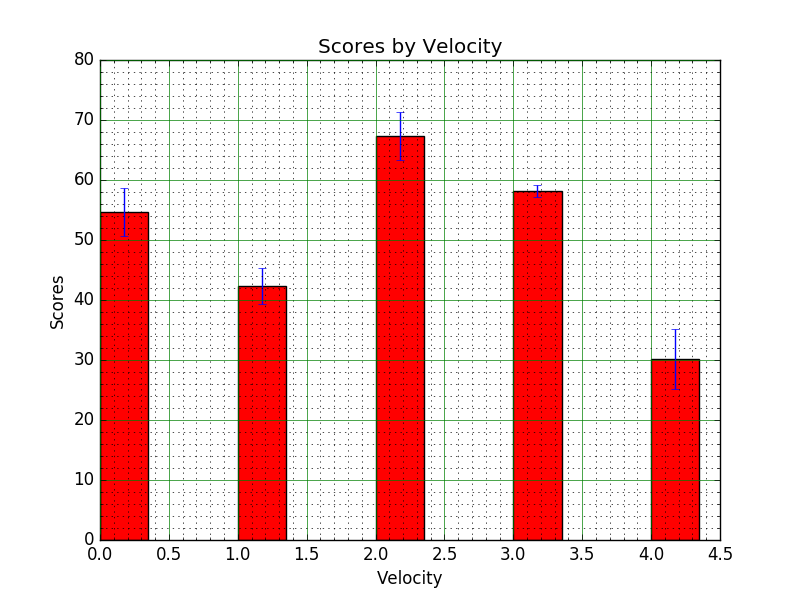﻿ Matplotlib Bar Chart: Create bar plots with errorbars on the same figure - w3resource# Matplotlib Bar Chart: Create bar plots with errorbars on the same figure

## Matplotlib Bar Chart: Exercise-12 with Solution

Write a Python program to create bar plots with errorbars on the same figure.

Sample Date
Mean velocity: 0.2474, 0.1235, 0.1737, 0.1824
Standard deviation of velocity: 0.3314, 0.2278, 0.2836, 0.2645

Sample Solution:

Python Code:

``````import numpy as np
import matplotlib.pyplot as plt
N = 5
menMeans = (54.74, 42.35, 67.37, 58.24, 30.25)
menStd = (4, 3, 4, 1, 5)
# the x locations for the groups
ind = np.arange(N)
# the width of the bars
width = 0.35
plt.bar(ind, menMeans, width, yerr=menStd, color='red')
plt.ylabel('Scores')
plt.xlabel('Velocity')
plt.title('Scores by Velocity')
# Turn on the grid
plt.minorticks_on()
plt.grid(which='major', linestyle='-', linewidth='0.5', color='green')
plt.grid(which='minor', linestyle=':', linewidth='0.5', color='black')
plt.show()
```
```

Sample Output:Python Code Editor: